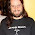## Sunday, November 18, 2012

### Calculus: Anti-derivatives and Area Under a Curve

The textbook we use (Briggs), and I think most of the textbooks I've used in the past (including Stewart and Thomas), introduce anti-derivatives before area under a curve. So they show students the notation for indefinite integrals before showing them the notation for definite integrals. I think this is a BIG mistake.

Here's what happens...
∫ f(x) dx (aka indefinite integral) means find all functions F(x) so that F'(x)=f(x).
(Why does it use that funny symbol? Why does it have that dx part at the end? Hard to explain without referencing a connection that hasn't been made yet, isn't it?)

And then we start thinking about areas under curves and use a notation that's almost the same.
12 f(x) dx (aka definite integral) means find the area which is between f(x) and the x-axis (area below the axis counts as negative), and between x=1 and x=2.

Seeing almost identical notation and names, we're going to assume that these two act the same in some ways. Students are going to expect anti-derivatives, even though it's area we're talking about here. So it's not much surprise when the Fundamental Theorem of Calculus tells us that to find area we can use anti-derivatives.

Wait! That should be a surprise. It's kind of amazing, isn't it? Derivatives give us slope. Why would going backwards in that process give us area?! Seems to me that's a big one we need to meditate on for a while.

This semester I knew I wanted to connect the new ideas with the position, velocity, and acceleration problems, so I introduced anti-derivatives first. And, I showed the indefinite integral symbol. Oops! I shouldn't have. If I had held off, I believe the meaning of the definite integral would have taken hold better in my student's minds.

Until this semester, I've followed the textbook pretty closely, so my way around this problem has been to introduce the 'Area Function' without using this notation. I found this idea/project in a book put out by the MAA.  I've revised it a lot over the years, but the original author, Charles Jones (of Grinnell College) still deserves credit for getting me started in this direction. (I wish I could figure out how to thank him personally, but he doesn't seem to be at Grinnell College these days, and google gives me lots of people with that name.)

I've put a pdf of the project here. If you'd like my Word file, just email me (mathanthologyeditor on gmail).

We've started that project, and it's going well enough, but I realized that if I hadn't introduced the indefinite integral, we'd be better off. Next semester I'll get that right.

Tomorrow we wrap up the project, and I clarify the implications of the Fundamental Theorem. Cool stuff!

1.This makes so much sense. The area function is very concrete and a much better point of departure for introducing integration.

I also agree that the unfamiliar notation and terminology is disconcerting. It can help to "demystify" it if you give students some helpful ways to associate meaning with those terms so they "own" them.

Your "area function" first approach lends itself very naturally to that, since you can talk about the integration symbol being just a fancy form of "S" for summation (and approximating areas obviously involves summing up many smaller areas with widths of dx).

And the word "integrate" also has helpful etymological associations, since the word literally means "to render something whole" and you are literally putting many different puzzle pieces together to compute the area of the whole when you integrate.

2.I like to start out by using F(x) as the symbol for the antiderivative of f(x), and the integral sign for the area. Then the fundamental theorem is really exciting and nonobvious! I certainly remember in my first calculus class, seeing that theorem and thinking "So the integral of the function is the integral? What is this pointless thing?" and then the problems we were given for it all involved d/dt of the integral from a to t of f(x) dx, and with all those letters flying around the whole thing didn't make any sense.

Your project looks fantastic -- I love the way you start, introducing the notation and the concept and estimation without any heavy machinery involved. Then moving on to linear functions -- maybe a bit too big a leap? I might use y = 3 first, and then y = 2x, and then their sum, with the bonus of introducing another fact about integrals.

Then they get to the Fundamental Theorem in such a way that it seems almost natural, like they're discovering it for themselves!

I'm not sure how much they know about antiderivatives before this project -- I'd be curious to know a little more about where in your course this lands.

3.We can avoid using any symbol for anti=derivative at first, by offering students functions labeled f'(x), ansking asking them to find f(x). I think that's my preference. And doing this project has made me notice that the definite integral is an odd new function, in that it needs two inputs. Our area function avoids this by keeping the left side constant, so I like the F(x) notation for this, which clarifies that it's just another function.

I think you're right about starting off more gradually. Maybe I should make those modifications now, before I forget.

I had done a section like the textbooks do, on anti-derivatives, so they saw how polynomials work, and probably sine, cosine, and e^x. I think we had done simple chain rule problems too, like find y when y'=sin(2x).

I'm reading a very interesting open source textbook right now by Crowell, and he makes the connection between rate of change and area clear in the first chapter of the book. He's starting out with discrete change. It's an intriguingly different approach.